## Area and Perimeter of Composite Figures

Practice Unlimited Questions

#### 1. The figure below is made of two identical rectangles and two identical quadrants. Find the perimeter of the shaded part.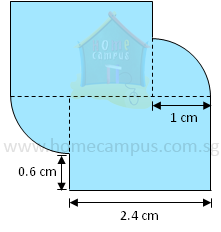= 1 cm + 0.6 cm
= 1.6 cm
Perimeter contributed by top rectangle
=  0.6 + 2.4 + 1.6
=  4.6 cm
Perimeter contributed by bottom rectangle
=  0.6 + 2.4 + 1.6
=  4.6 cm
=
 1 24
× 21× 3.14 × 1
=  1.57 cm
=
 1 24
× 21× 3.14 × 1
=  1.57 cm
=  Perimeter contributed by top rectangle
+  Perimeter contributed by left quadrant
+  Perimeter contributed by bottom rectangle
+  Perimeter contributed by right quadrant

=  4.6 + 1.57 + 4.6 + 1.57
=  12.34 cm

#### 2. The figure below shows two identical quadrants in two identical squares and a rectangle joining them to form a butterfly. Find the perimeter of the shaded butterfly.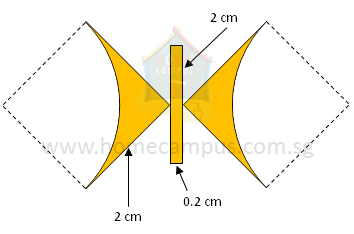Perimeter contributed by 1 square
=  2 + 2
=  4 cm
=
 1 14
× 21× 3.14 × 21
=  1.57 cm
Perimeter contributed by rectangle
=  0.2 + 2 + 0.2 + 2
=  4.4cm
=  2 × Perimeter contributed by 1 square
+  2 × Perimeter contributed by 1 quadrant
+  Perimeter contributed by rectangle

=  (2 × 4) + (2 × 3.14) + 4.4
=  8 + 6.28 + 4.4
=  18.68 cm

#### 3. Maddie joined 3 semicircles and a rectangle to form the shape of an umbrella. Find the area of the shaded umbrella.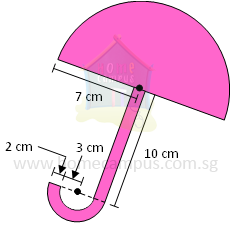Area of big semicircle
=
 1 12
×
 1122 17
× 71× 7
=  77 cm2
Area of medium semicircle
=
 1 2
× 3.14 × 5 × 5
=  39.25 cm2
Area of small semicircle
=
 1 2
× 3.14 × 3 × 3
=  14.13 cm2
Area of rectangle
=  10 × 2
=  20 cm2
=  Area of big semicircle
+  Area of rectangle
+  Area of medium semicircle
-  Area of small semicircle

=  77 + 20 + 39.25 − 14.13
=  122.12 cm2

#### 4. Find the area of the shaded heart formed by 2 semicircles and a square.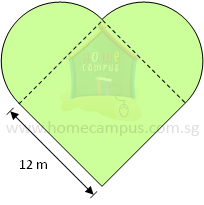= 12 cm ÷ 2
= 6 cm
Area of two semicircles
= Area of 1 circle
= 3.14 × 6 × 6
=  113.04 cm2
Area of square
=  12 × 12
=  144 cm2
=  Area of 2 semicircles
+  Area of square

=  113.04 + 144
=  257.04 cm2

#### 5. Find the area of the figure below formed by 2 identical three-quarter circles, 2 isosceles right-angled triangles and a square.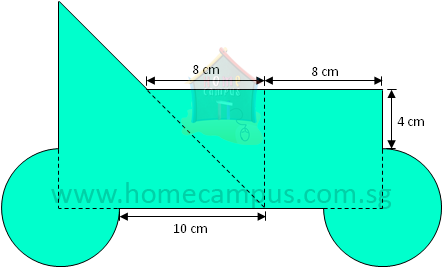= 8 cm - 4 cm
= 4 cm
Area of 1 three-quarter circle
=
 3 14
× 3.14 × 41× 4
=  37.68 cm2
Height of right isosceles triangle
= Base of right isosceles triangle
= 4 + 10 cm = 14 cm
Area of big isosceles right triangle
=
 1 12
× 147× 14
=  98 cm2
Area of small isosceles right triangle
=
 1 12
× 87× 8
=  32 cm2
Area of square
=  8 × 8
=  64 cm2
=  2 × Area of 1 three-quarter circle
+  Area of big isosceles triangle
+  Area of small isosceles triangle
+  Area of square

=  (2 × 37.68) + 98 + 32 + 64
=  75.36 + 98 + 32 + 64
=  269.36 cm2

#### 6. Find the area and perimeter of the shaded figure below.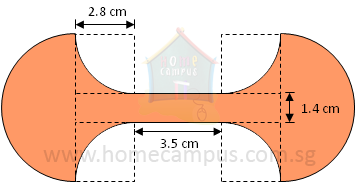Diameter of semicircle
= 2.8 + 1.4 + 2.8
= 7 cm
PERIMETER
Perimeter contributed by 1 semicircle
=
 1 12
×
 1122 17
× 71
=  11 cm
=
 1 14
× 21 ×
 1122 17
× 2.80.4
=  4.4 cm
Perimeter contributed by rectangle
=  3.5 + 3.5 cm
=  7 cm
=  2 × Perimeter contributed by 1 semicircle
+  4 × Perimeter contributed by 1 quadrant
+  Perimeter contributed by rectangle

=  (2 × 11) + (4 × 4.4) + 7
=  22 + 17.6 + 7
=  46.6 cm
= 7 cm ÷ 2
= 3.5 cm
AREA
Area of 1 semicircle
=
 1 12
×
 1122 17
× 3.50.5 × 3.5
=  19.25 cm2
Area of 1 square
=  2.8 × 2.8
=  7.84 cm2
=
 1 14
×
 22 17
× 2.80.7 × 2.80.4
=  6.16 cm2
Length of rectangle
= 3.5 + 2.8 + 2.8
= 9.1 cm
Area of rectangle
=  9.1 × 1.4
=  12.74 cm2